# Differential-geometric structure

(diff) ← Older revision | Latest revision (diff) | Newer revision → (diff)
Jump to: navigation, search

One of the fundamental concepts in modern differential geometry including the specific structures studied in classical differential geometry. It is defined for a given differentiable manifold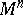as a differentiable section in a fibre space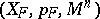with baseassociated with a certain principal bundle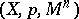or, according to another terminology, as a differentiable field of geometric objects on. Hereis some differentiable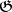-space whereis the structure Lie group of the principal bundleor, in another terminology, the representation space of the Lie group.

Ifis the principal bundle of frames in the tangent space to,is some closed subgroup in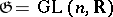, andis the homogeneous space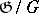, the corresponding differential-geometric structure onis called a-structure or an infinitesimal structure of the first order. For example, ifconsists of those linear transformations (elements of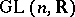) which leave an-dimensional space ininvariant, the corresponding-structure defines a distribution of-dimensional subspaces on. Ifis the orthogonal group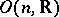— the subgroup of elements ofwhich preserve the scalar product in—, then the-structure is a Riemannian metric on, i.e. the field of a positive-definite symmetric tensor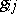. In a similar manner, almost-complex and complex structures are special cases of-structures on. A generalization of the concept of a-structure is an infinitesimal structure of order,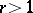(or-structure of a higher order); hereis the principal bundle of frames of the orderon, andis a closed subgroup of its structure group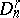.

All kinds of connections (cf. Connection) are important special cases of differential-geometric structures. For instance, a connection in a principal bundle is obtained if the role ofis played by the spaceof some principal bundle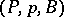, and the-structure onis the distribution of-dimensional,, subspaces complementary to the tangent spaces of the fibres which is invariant with respect to the action onof the structure group of the bundle. Connections on a manifoldare special cases of differential-geometric structures on, but more general ones than-structures on. For instance, an affine connection on, definable by a field of connection objects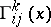, is obtained as the differential-geometric structure onfor whichis the principal bundle of frames of second order,is its structure group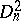, and the representation spaceofis the spacewith coordinates, where the representation is defined by the formulas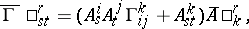whereare the coordinates of an element of the group, and. In the case of a projective connection onone deals with a certain representation of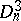in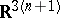, while in cases of connections of a higher order, one deals with representations of. By this approach the theory of differential-geometric structures becomes closely related to the theory of geometric objects (Cf. Geometric objects, theory of).

How to Cite This Entry:
Differential-geometric structure. Encyclopedia of Mathematics. URL: http://encyclopediaofmath.org/index.php?title=Differential-geometric_structure&oldid=15582
This article was adapted from an original article by Ãœ. Lumiste (originator), which appeared in Encyclopedia of Mathematics - ISBN 1402006098. See original article